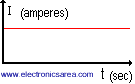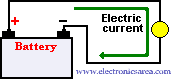# The Continuous current or Direct current

Continuous current or direct current (DC), is the result of the flow of electrons (negative charge) in a conductor (most of the time, usually wires of copper) that goes only in one direction. If we have a battery, the electric current will flow from the negative to the positive terminal (one direction) The load in this case is a light bulb. The charge of an electron is very small.

The unit of charge used is the Coulomb (much bigger than the charge of an electron).

1 Coulomb = the charge of 6 280 000 000 000 000 000 electrons or in scientific notation: 6.28 x 1018 electrons.The continuous current or direct current can change its magnitude
butit can not change its direction with time.

To be consistent with our diagram and with the existent convention, the current flows from the positive terminal to the negative terminal. This seems to be the opposite of what we say before.

What happens is that when an electron is passing throughout the conductor, leaves a hole (positive) that it is occupied at the same time by another electron that leaves another hole and so forth, generating a series of holes that travel in the opposite direction of the flow of the electrons.

The electric current is the amount of charge that flow through the lamp in one second, then:
Electric current = Amount of charge (coulombs)/time(seconds) or I = Q/T. If the charge that runs through the lamp is 1 coulomb per second, the current is 1 ampereFor example: If there is a charge of 14 coulombs pasing throughout the light bulb per second, then the current will be: I = Q/T = 14 Coulombs/1 second = 14 amperes

The electric current is measured in Amperes (A) and the electronic circuits are generally measured in miliAmperes (mA) or microAmperes (uA). See the following conversions.

1 miliampere (mA) = 0.001 Amperes (A)
1 microAmpere (uA) = 0.000001 Amperes (A)

•
•
•
•
•
•
•
•
•
•
•
•
•
•
•
•
•
•